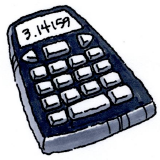### Home > CC3 > Chapter 2 > Lesson 2.1.7 > Problem2-71

2-71.

CALCULATOR CHECK

Use your scientific calculator to compute the value of each expression in the left-hand column below. Match each result to an answer in the right-hand column.1.  $−3 + 16 − \left(−5\right)$

2. $\left(3 − 5\right)\left(6 + 2\right)$

3. $17\left(−23\right) + 2$

4. $5 − \left(3 − 17\right)\left(−2 + 25\right)$

5. $\left(−4\right)\left(−2.25\right)\left(−10\right)$

6. $-1.5-2.25-\left(-4.5\right)$

7. $\frac{4-5}{-2}$

1.  $−16$

2. $327$

3. $0.5$

4. $18$

5. $−90$

6. $0.75$

7. $−389$

Remember to be cautious when entering negative signs.
A few matches have been made below, so that you can check some of your work.

$\text{a.}\ \Rightarrow\ 4.$

$\text{f.}\ \Rightarrow\ 6.$

$\text{g.}\ \Rightarrow\ 3.$